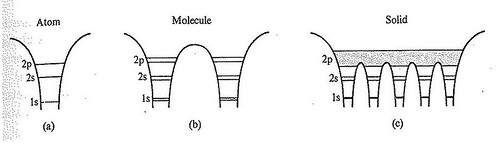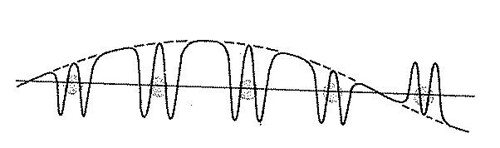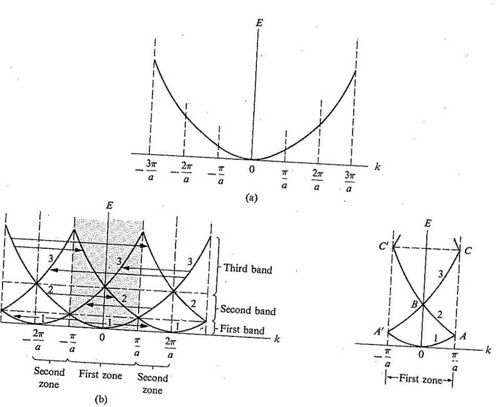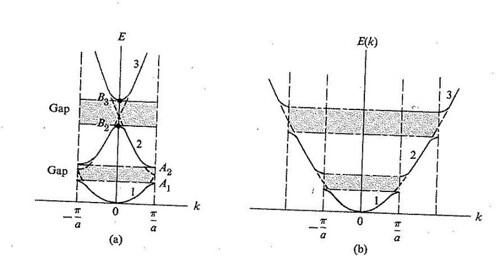# Energy bands in solids and their calculations

$$\newcommand{\vecs}{\overset { \rightharpoonup} {\mathbf{#1}} }$$ $$\newcommand{\vecd}{\overset{-\!-\!\rightharpoonup}{\vphantom{a}\smash {#1}}}$$$$\newcommand{\id}{\mathrm{id}}$$ $$\newcommand{\Span}{\mathrm{span}}$$ $$\newcommand{\kernel}{\mathrm{null}\,}$$ $$\newcommand{\range}{\mathrm{range}\,}$$ $$\newcommand{\RealPart}{\mathrm{Re}}$$ $$\newcommand{\ImaginaryPart}{\mathrm{Im}}$$ $$\newcommand{\Argument}{\mathrm{Arg}}$$ $$\newcommand{\norm}{\| #1 \|}$$ $$\newcommand{\inner}{\langle #1, #2 \rangle}$$ $$\newcommand{\Span}{\mathrm{span}}$$ $$\newcommand{\id}{\mathrm{id}}$$ $$\newcommand{\Span}{\mathrm{span}}$$ $$\newcommand{\kernel}{\mathrm{null}\,}$$ $$\newcommand{\range}{\mathrm{range}\,}$$ $$\newcommand{\RealPart}{\mathrm{Re}}$$ $$\newcommand{\ImaginaryPart}{\mathrm{Im}}$$ $$\newcommand{\Argument}{\mathrm{Arg}}$$ $$\newcommand{\norm}{\| #1 \|}$$ $$\newcommand{\inner}{\langle #1, #2 \rangle}$$ $$\newcommand{\Span}{\mathrm{span}}$$$$\newcommand{\AA}{\unicode[.8,0]{x212B}}$$

When I started to think about it, I felt that the main problem was to explain how the electrons could sneak by all the ions in a metal. By straight Fourier analysis, I found to my delight that the wave diffracted from the plane wave of free electrons only by a periodic modulation. -F. Bloch

No property of solids varies as widely as their ability to conduct electric current and we can see this by the striking difference in resistivity between a good conductor and a good insulator. The electrical resistivity of a pure metal may be as low as 10-12 ohm-cm at a temperature of 1 K, apart from the possibility of superconductivity. The resistivity of a good insulator may be as high as 1022 ohm-cm. This range of 1032 may be the widest of any common physical property of solids. The existence of electron energy bands in solids makes it possible to understand this remarkable span.$$^{}$$

We can begin by considering the energy levels of the individual atoms as they are brought together. When the atoms are far apart, the energy of a particular level is the same for each atom. The electrons of a single free-standing atom occupy atomic orbitals, which form a discrete set of energy levels.The allowed energy levels in an isolated atom are often far apart. For example, in Hydrogen, the lowest possible allowed energy ($$E_1$$ = -13.6 eV) is 10.2 eV below the next lowest ($$E_2$$ = -13.6 eV/4 = -3.4 eV)$$^{}$$.

As the atoms are brought closer together, each of the energy level for each atom changes because of the influence of the other atom. As a result, the level split into two levels of slightly different energies for the two-atom system as in the case of hydrogen molecule ion $$H_2^+$$$$^{}$$. The reason is as follows, when the two atoms are far apart, say in the case of two Li atoms, influence of one atom on an electron in the other atom is very small, and may be treated as perturbation. In this approximation, the unperturbed levels 1s, 2s etc., are each doubly degenerate; because the electrons in a 1s level, for instance, may occupy that level in either atom; and since there are two atoms, the energy is thus doubly degenerate. This degeneracy is strictly valid only if the interaction between the atoms is neglected entirely. When this interaction is included, the double degeneracy is lifted, and each level is split into its two sublevels$$^{}$$. The molecular orbitals corresponding to these sublevels are usually taken to be the symmetric and antisymmetric combinations of the corresponding atomic orbitals, as in the case of $$H_2^+$$$$^{}$$.

Each molecular level can accommodate at most two electrons, of opposite spins, according to the exclusion principle. The $$Li_2$$ molecule has six electrons; four occupy the 1s molecular doublet, and the other two lower levels of the 2s doublet. Thus the amount of splitting depends strongly on the internuclear distance of the two atoms in the molecule. The closer the two nuclei, the stronger the perturbation and larger the splitting. The splitting also depends on the atomic orbital: the splitting of the 2p level is larger than that of the 2s level, which is larger than that of the 1s level. The reason is that the radius of the 1s orbital, for instance, is very small, and the orbital is therefore tightly bound to its own nucleus. It is not greatly affected by the perturbation. The same is not true for the 2s and 2p orbitals, which have larger radii and are only loosely bound to their own nuclei. It follow that, the higher the energy, the greater the splitting incurred$$^{}$$.

If we bring three atoms close together, a particular energy level splits into three separate levels of slightly different energies. If several atoms are brought together into a molecule, their atomic orbitals split and produce a number of molecular orbitals proportional to the number of atoms. Maria in her term project$$^{}$$ discussed about the atomic and molecular orbitals. Figure $$\PageIndex{1}$$ shows the evolution of the energy spectrum of Li from an atom (a), to a molecule (b), to a solid (c).Figure $$\PageIndex{1}$$ The evolution of the energy spectrum in Li from an atom (a), to a molecule (b), to a solid (c).

When a large number of atoms (of order 1023 or more) are brought together to form a solid, the number of orbitals becomes exceedingly large, and the difference in energy between them becomes very small, so the levels may be considered to form continuous bands of energy rather than the discrete energy levels of the atoms in isolation. Within an energy band, energy levels are so numerous as to be a near continuum$$^{}$$. However, some intervals of energy contain no orbitals i.e., the forbidden energy levels, no matter how many atoms are aggregated, forming band gaps. The bands may be widely separated in energy, they may be close together, or they may even over lap, depending on the kind of atom and the type of bonding in the solid and thus can explain the fundamental properties of the solids. Roald Hoffmann's article, "How chemistry and physics meet in the solid state" gives the sense of the marvelous electronic properties of the solid state and tries to explain the chemists the language of solid state physics, of band structures$$^{}$$.

## The Bloch Theorem

The behavior of an electron in a crystalline solid is determined by studying the appropriate Schrödinger equation. This may be written as

\begin{align} \left(-\frac{\hbar^2}{2m}\nabla^2 + V(\mathbf{r}) \right )\Psi(\mathbf{r}) = E\Psi(\mathbf{r}) \end{align} \label{1}

where $$V(r)$$ is the crystal potential seen by the electron, and $$\Psi(\mathbf{r}$$ and $$E$$ are respectively, the state function and the energy of this electron. The potential $$V(r)$$ includes the interaction of the electron with all atoms in the solid, as well as its interaction with other electrons. $$V(r)$$ is periodic and has the translational symmetry as the lattice, that is,

\begin{align} V(\mathbf{r}+\mathbf{R})=V(\mathbf{r}) \end{align} \label{2}

where $$\mathbf{R}$$ is the lattice vector. Such potential is shown schematically in Figure $$\PageIndex{2}$$. According to the Bloch theorem, the solution of Equation (1) for a periodic potential $$V(r)$$ has the form

\begin{align} \Psi_\mathbf{k} (\mathbf{r}) = e^{i\mathbf{k}.r} u_\mathbf{k} (\mathbf{r}) \end{align} \label{3}

where the function $$u_\mathbf{k} (\mathbf{r})$$ has the same translational symmetry of the lattice, that is,

\begin{align} u_\mathbf{k} (\mathbf{r}+\mathbf{R})=u_\mathbf{k} (\mathbf{r}) \end{align} \label{4}

The vector k is a quantity related to the momentum of the particle. It is possible to write the solution of Equation (1) as

\begin{align} \Psi(\mathbf{r})=f(\mathbf{r})u(\mathbf{r}) \end{align} \label{5}

where $$u(\mathbf{r})$$ is periodic, as in Equation (4), and the function $$f(\mathbf{r})$$ is to be determined. However, since the potential $$V(\mathbf{r})$$ is periodic, it requires that all observable quantities associated with the electron are also periodic. In particular the quantity $$|\Psi (\mathbf{r})|^2$$ (physically observable), which gives the electron probability, must also be periodic. This imposes the following conditions of $$f(\mathbf{r})$$:

\begin{align} |f(\mathbf{r}+\mathbf{R})|^2=|f(\mathbf{r})|^2 \end{align} \label{6}Figure $$\PageIndex{2}$$ The crystal potential seen by the electron.

The only function which satisfies the requirements for all R's is one of the exponential form $$e^{i\mathbf{k}.r}$$. This demonstrates that the solution of the Schrödinger equation has the Bloch form.The state function of the $$\Psi_k$$ of the form of Equation (3), known as Bloch function, has interesting properties.

1. It has the form of a traveling plane wave, as represented by the factor $$e^{i\mathbf{k}.r}$$, which implies that the electron propagates through the crystal like a free particle. The effect of the function $$u_\mathbf{k}(\mathbf{r})$$ is to modulate this wave so that the amplitude oscillates periodically form one cell to the next, as shown in Figure 3, but this does not affect the basic character of the state function, which is that of a traveling wave. If the electrons were indeed entirely free, the state function $$\Psi_k$$ should be given by (1/ $$V^{0.5}$$), that is, the function $$u_\mathbf{k}(\mathbf{r})$$ is a constant. But the electron is not free, since it interacts with the lattice, and this interaction determines the special character of the periodic function $$u_\mathbf{k}$$.
2. Because the electron behaves like a wave of vector $$\mathbf{k}$$, it has a de Broglie wavelength and hence a momentum and is the crystal momentum of the electron.
3. The Bloch function $$\mathbf{\Psi}_{\mathbf{k}}$$ is a crystal orbital, as it is delocalized throughout the solid, and not localized around any particular atom. Thus the electron is shared by the whole crystal$$^{[2,6]}$$.Figure $$\PageIndex{3}$$ The Bloch function as wave. The smooth curve represents the wave $$e^{i\mathbf{k}.r}$$ which is modulated by the atomic like function $$u_\mathbf{k}(\mathbf{r})$$.

## Energy Bands

The energy spectrum result from solving the Equation (1) have large number of solutions, giving discrete energies $$E_{1,\mathbf{k}}$$, $$E_{2,\mathbf{k}}$$ etc., for each value of k and is shown in Figure $$\PageIndex{4}$$. Since these energies depend on $$\mathbf{k}$$, they vary continuously as $$\mathbf{k}$$ is varied over its range of values. Each level leads to a energy band and shall write the energy eigenvalue as $$E_{n}(\mathbf{k})$$, and refer to the subscript n as band index. The number of bands is large and only the lowest ones are occupied by electrons. Each band covers a certain energy range, extending from the lowest to the highest value it takes when plotted in $$\mathbf{k}$$ space. The energy intervals between the bands constitute the energy gaps, which are forbidden energies that cannot be occupied by electrons. As $$\mathbf{k}$$ is a vector quantity, a diagram such as Figure $$\PageIndex{4}$$ is a plot of energy bands in only one direction in $$\mathbf{k}$$ space. If the bands were plotted in a different $$\mathbf{k}$$ direction, they will change. A complete representation of the bands therefore requires one to specify the energy values through out the $$\mathbf{k}$$ space. Often this is accomplished, at least partially, by drawing the energy contours in $$\mathbf{k}$$ space for various bands. But the bands obey certain symmetry conditions and restricts our consideration to relatively small regions in $$\mathbf{k}$$ space.

The dispersion relation between the energy and momentum of electrons can best be described in reciprocal space. It turns out that for crystalline structures, the dispersion relation of the electrons is periodic, and that the Brillouin zone is the smallest repeating space within this periodic structure. For an infinitely large crystal, if the dispersion relation for an electron is defined throughout the Brillouin zone, then it is defined throughout the entire reciprocal space$$^{}$$. The topic of Brillouin zones and the band symmetry in $$\mathbf{k}$$ space is explained in detail by Ching-Chang in his term paper$$^{}$$.Figure $$\PageIndex{4}$$ Energy bands and gaps.

The parabolic representation of the dispersion curve for a free particle, $$E=\dfrac{\hbar ^2}{2m_0}$$ for the same particle in empty lattice model, showing translational symmetry and the various bands and the dispersion curve for the first zone is shown in Figure $$\PageIndex{5}$$. Dispersion curve in the nearly free electron model in the reduced scheme zone showing only the first Brillouin zone and the same dispersion curve in the extended zone scheme is shown in Figure $$\PageIndex{6}$$.Figure $$\PageIndex{5}$$ (a) Dispersion curve for a free particle, (b) for the same particle in empty lattice model, showing the translational symmetry and various bands, (c) dispersion curve in empty lattice model in first zone only.Figure $$\PageIndex{6}$$ Dispersion curve in near free electron model in first Brillouin zone by reduced zone scheme and the same curves in extended zone scheme.

Any solid has a large number of bands. In theory, it can be said to have infinitely many bands (just as an atom has infinitely many energy levels). However, all but a few lie at energies so high that any electron that reaches those energies escapes from the solid. These bands are usually disregarded. Bands have different widths, based upon the properties of the atomic orbitals from which they arise. Also, allowed bands may overlap, producing (for practical purposes) a single large band. Figure $$\PageIndex{7}$$, shows a simplified picture of the bands in a solid that allows the three major types of materials to be identified: metals, semiconductors and insulators.Figure $$\PageIndex{7}$$ Simplified diagram of the electronic band structure of metals, semiconductors, and insulators.

Metals contain a band that is partly empty and partly filled regardless of temperature. Therefore they have very high conductivity. The lowermost, almost fully occupied band in an insulator or semiconductor is called the valence band by analogy with the valence electrons of individual atoms. The uppermost, almost unoccupied band is called the conduction band because only when electrons are excited to the conduction band can current flow in these materials. The difference between insulators and semiconductors is only that the forbidden band gap between the valence band and conduction band is larger in an insulator, so that fewer electrons are found there and the electrical conductivity is lower. Because one of the main mechanisms for electrons to be excited to the conduction band is due to thermal energy, the conductivity of semiconductors is strongly dependent on the temperature of the material. Fermi energy concept have been discussed by Coons in the term project$$^{}$$.

The bandgap of a semiconductor is always one of two types, a direct bandgap or an indirect bandgap. The minimal-energy state in the conduction band, and the maximal-energy state in the valence band, are each characterized by a certain $$\mathbf{k}$$-vector in the Brillouin zone. If the $$\mathbf{k}$$-vectors are the same, it is called a direct gap. If they are different, it is called an indirect gap.

This band gap is one of the most useful aspects of the band structure, as it strongly influences the electrical and optical properties of the material. Electrons can transfer from one band to the other by means of carrier generation and recombination processes. The band gap and defect states created in the band gap by doping can be used to create semiconductor devices such as solar cells, diodes, transistors, laser diodes, and others$$^{}$$.

## References

1. Kittel, Charles. “Introduction to Solid State Physics”, Chapter 7. John Wiley & Sons, Inc., (2005).
2. Omar, M. Ali. “Elementary Solid State Physics”, Chapter 5. Pearson Education, (2007).
3. http://en.Wikipedia.org/wiki/Electro...band_structure
4. Hoffmann, R. How chemistry and physics meet in the Solid state, Angew. Chem. Int. Ed. Engl. 26 (1987) 846-878.
5. Kohanoff, J. Electronic Structure Calculations for Solids and Molecules, Cambridge University Press, (2006).